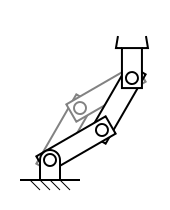[Documentation] [TitleIndex] [WordIndex]## pr2_arm_ik_action

pr2_arm_ik_action action is uses the joint_trajectory_action to move the wrist roll link to a desired pose.

### Action Goal

<~arm>_arm_ik/goal (pr2_common_action_msgs/ArmMoveIKGoal)
• This is the desired pose of the wrist roll link.

### Action Result

<~arm>_arm_ik/result (pr2_common_action_msgs/ArmMoveIKResult)
• This returns the location where the plug was found in the gripper.

### Actions Called

<~arm>_arm_controller/<~joint_trajectory_action> (joint_trajectory_action)
• This action moves the arm to a joint configuration.

### Parameters

~arm (string, default: r)
• Which arm to control (l or r).
~joint_trajectory_action (string, default: r_arm_controller/joint_trajectory_action)
• The namespace of the joint trajectory action to send trajectories to.
~free_angle (int, default: 2)
• Since the PR2 arms are redundant (they have 7 DOF), there are an infinite number of joint space configurations that satisfy a given Cartesian end effector pose. The ik solver will compute a joint space solution solution for every value of the "free angle" joint, and the valid solution that is closest to the seed configuration in the goal is chosen.
• The search step size for the "free angle" joint.
~ik_timeout (double, default: 5.0 sec)
• The timeout on the ik solver. The action will fail if no valid solution is found withing this duration.

Example launch file :

```<launch>
<!-- ik action -->
<node pkg="pr2_arm_ik_action" type="arm_ik" name="r_arm_ik" output="screen">
<param name="joint_trajectory_action" value="joint_trajectory_generator" />
<param name="arm" value="r" />
<param name="free_angle" value="2" />
<param name="search_discretization" value="0.01" />
<param name="ik_timeout" value="5.0" />
</node>

<!-- Trajectory generator -->
<node pkg="joint_trajectory_generator" type="joint_trajectory_generator" output="screen"
name="joint_trajectory_generator" ns="r_arm_controller" >
<param name="max_acc" value="2.0" />
<param name="max_vel" value="2.5" />
</node>
</launch>```

2020-02-22 13:02